Home / Tradeshift Documentation / Tradeshift Partner Apps / Babelway / Chapter 4. Channels

## Sub-formulas

13180 0 Created on 2021-01-19 07:45:58; Last updated on 2022-06-25 19:00:41

Sub-formulas are parts of formulas that you can name and reuse later.

Sub-formulas are another way to simplify the writing of very long formulas.

• When you work on this part of the formula, you only see this part (instead of the whole formula), and do not have to identify this part again and again inside the whole formula.

• When you work on the main formula, it is much smaller and readable, as all complex parts have been factorized and are just replaced by a reference to the sub-formula.

Sub-formulas also allow you to share common parts of long formulas across different formulas. If you need to change this part, you will only have to change it once, and all formulas using it will be automatically updated.

#### Example

As an example, let's imagine that you have to make some complex calculations to calculate a date and time, then put the date in one field, and the time in another field.

```[DeliveryDate] = formatDateTime(addDays(parseDateTime([yourDate], 'yyyyMMddhhmm'), if ([productType]=1 or [productType]=2) then 3 else 2), 'dd/MM/yyyy')
[DeliveryTime] = formatDateTime(addDays(parseDateTime([yourDate], 'yyyyMMddhhmm'), if ([productType]=1 or [productType]=2) then 3 else 2), 'hh:mm:ss')```

By using a sub-formula you could factorize the common part and just let the outer formulas deal with the selection of date or time in complex formulas.

```[DeliveryDate] = formatDateTime([DeliveryDateTime], 'dd/MM/yyyy')
[DeliveryTime] = formatDateTime([DeliveryDateTime], 'hh:mm:ss')
[DeliveryDateTime] = addDays(parseDateTime([yourDate], 'yyyyMMddhhmm'), if ([productType]=1 or [productType]=2) then 3 else 2)
```

The new version is much more readable. If you still find it too complex, you could even go further, and separate the calculation of [DeliveryDateTime] in 2 parts.

```[DeliveryDate] = formatDateTime([DeliveryDateTime], 'dd/MM/yyyy')
[DeliveryTime] = formatDateTime([DeliveryDateTime], 'hh:mm:ss')
[DaysNeededToDeliver] = if ([productType]=1 or [productType]=2) then 3 else 2
```

#### Execution

The result of the execution of the formulas will always be exactly the same whether you factorize some parts in sub-formulas or not. Using sub-formulas is a way of writing your formula easier. It is always completely equivalent to the fully expanded formula.

To illustrate, let's take the following complete formula, whose result will be '1 2'

`concat(nextCounterValue('invoice'), ' ', nextCounterValue('invoice'))`

If you decide to write it using a sub-formula, the result will still be exactly the same : '1 2', because nextCounterValue will still be evaluated twice, exactly like in the previous formula.

```concat([mySubFormula], ' ', [mySubFormula])
[mySubFormula] = nextCounterValue('invoice')```

#### Create a sub-formula

You can find sub-formulas at the top of the output tree.

To create a new sub-formula, just open the section, click on the '...' at the bottom of the section, and choose the name that you want to give to the sub-formula.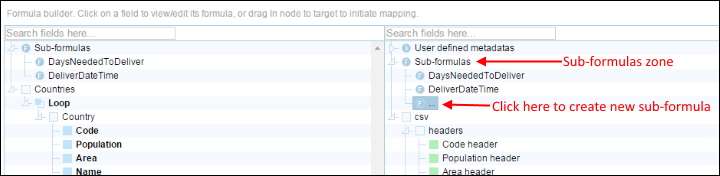Create sub-formula (1)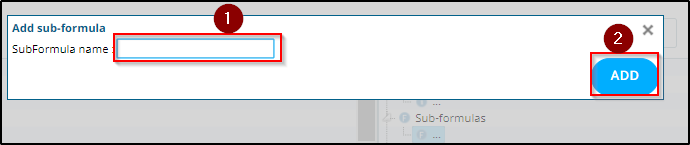Create sub-formula (2)

#### Define a sub-formula

Sub-formulas can be defined the same way as any other output field. See the sections about how to map nodes or how to edit formulas for more details.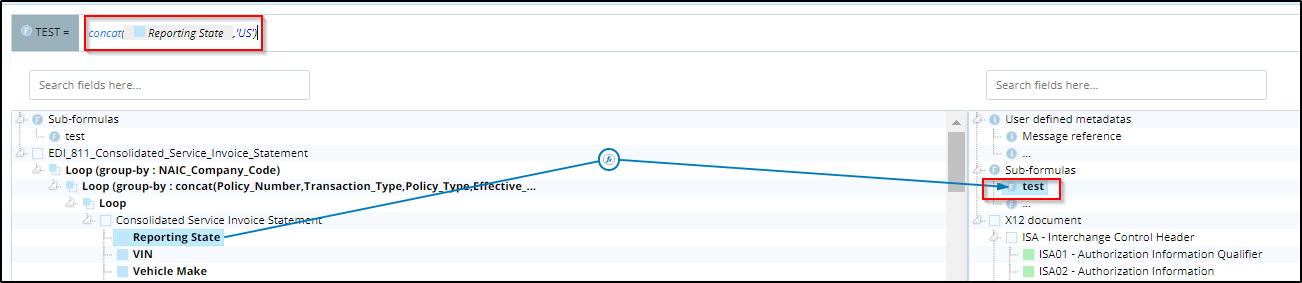Define a sub-formula

#### Use a sub-formula

Sub-formulas can be used the same way as any other input field. To make this possible, all sub-formulas are also available at the top of the tree with your incoming message. You can really see them as "virtual input fields", or "calculated input fields".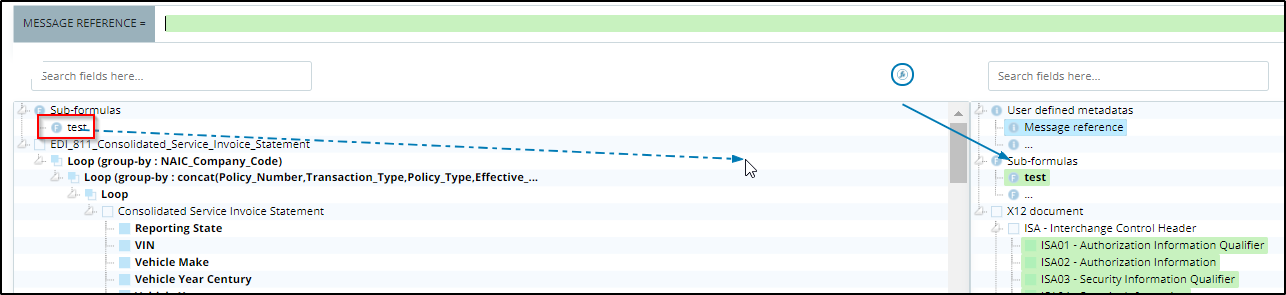Use a sub-formula

In your formulas, sub-formula references can be easily recognized : they are drawn like references to input fields, but with a purple text.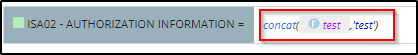Sub-formula reference in a formula

#### Global overview

When you have many nested sub-formulas, or user-functions, you can always have a global overview of your formula by using the "Copy-paste version".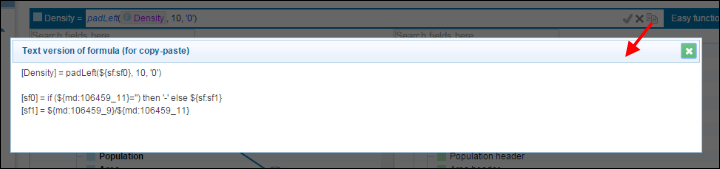Sub-formula global view

#### Delete a sub-formula

To delete a sub-formula, use the action at the right of the formula bar.

The sub-formula will be deleted, and all references to this sub-formula will be inlined, leading to the situation you would have had by writing all your formulas without using this sub-formula.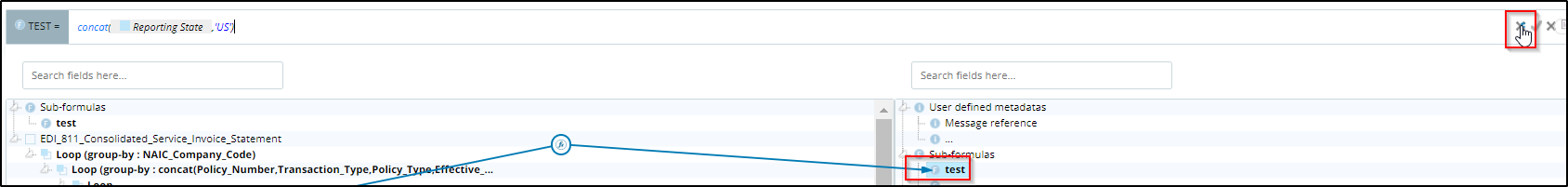Delete sub-formula

﻿﻿